# Arc Suppression Coil or Petersen Coil

There is always a significant charging current flowing from conductor to earth in underground high voltage and medium voltage power network. This is because of dielectric insulation between earth and conductor in the underground cables. During an earth fault in any phase, in a 3 phase such system, the charging current of the system ideally becomes three times more than rated charging current per phase. This larger charging current restrikes and passes to ground through faulty point and causing arcing there. To minimize the large capacitive charging current during earth fault, one inductive coil is connected from star point to earth. The current created in this coil during fault is opposite of cable charging current at same instant, thus neutralizes the charging current of the system during. This coil of suitable inductance is known as Arc Suppression Coil or Petersen Coil.

The voltages of a three phase balanced system is shown in figure – 1.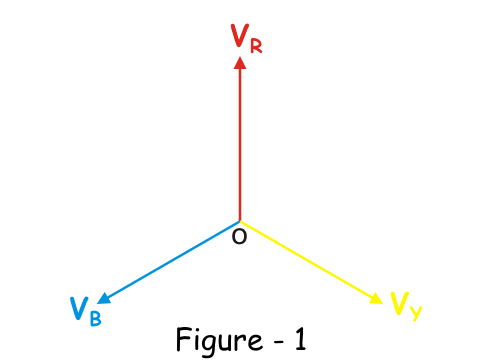In underground high voltage and medium voltage cable network, there is always a capacitance between conductor and earth in each phase. Because of that there is always a capacitive current from phase to earth. In each phase the capacitive current leads the corresponding phase voltage by 900 as shown in figure – 2.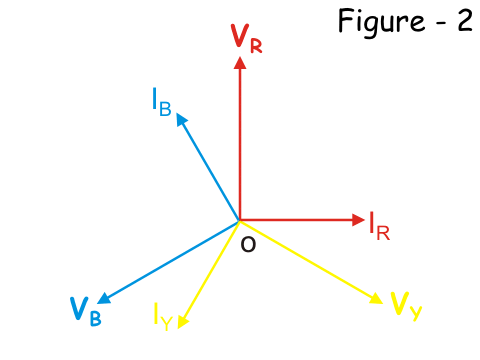Now suppose there is an earth fault at yellow phase of the system. Ideally, the voltage of yellow phase that is yellow phase to ground voltage becomes zero. Thus, null point of the system is shifted at the tip of the yellow phase vector, as shown in the figure-3, below. As a result, the voltage in healthy phases (red and blue) becomes &sqrt;3 times of the original.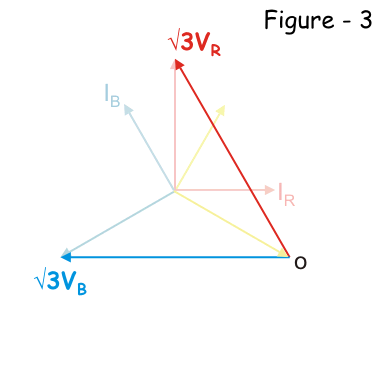Naturally, the corresponding capacitive current in each healthy phase (red and blue) becomes &sqrt;3 of the original as shown in figure-4, below.The vector sum that is resultant of these two capacitive current now will be 3I, where I is taken as rated capacitive current per phase in the balanced system. That means, at healthy balanced condition of the system, IR = IY =
IB = I.

This is illustrated in the figure- 5 below,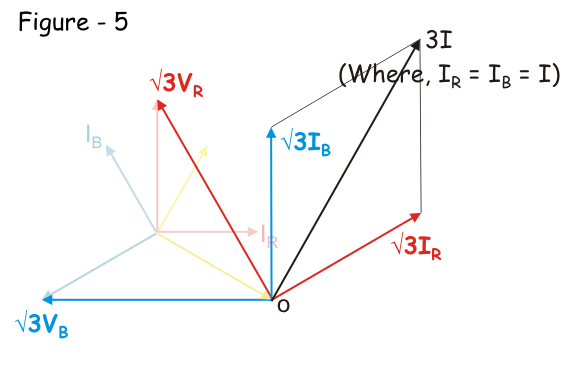This resultant current then flows through the faulty path to the earth as shown below.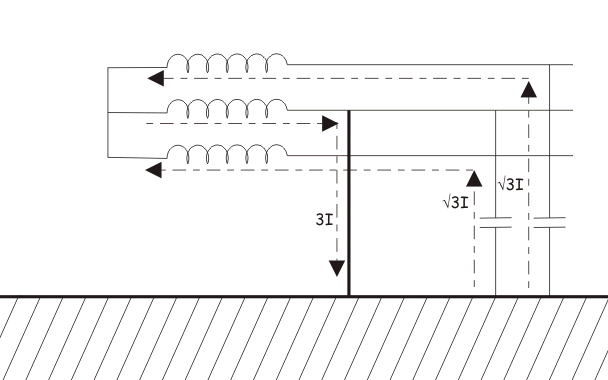Now, if we connect one inductive coil of suitable inductance value (generally iron core inductor is used) between star point or neutral point of the system and ground, the scenario will be entirely changed. At faulty condition, the current through the inductor just equal and opposite in magnitude and phase of that of capacitive current through the faulty path. The inductive current also follow the faulty path of the system. The capacitive and inductive current cancels each other at the faulty path, hence there will not be any resultant current through the faulty path created due to capacitive action of the underground cable. The ideal situation is illustrated in the figure below.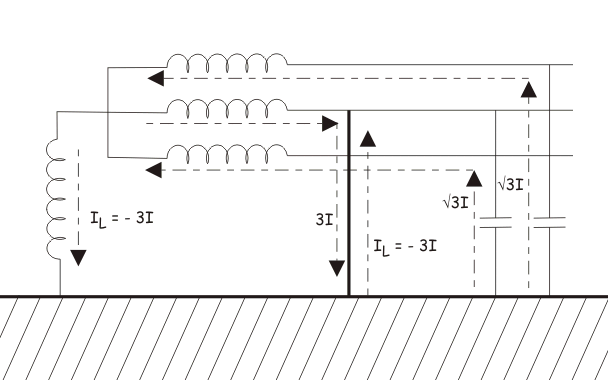This concept was first implemented by W. Petersen in 1917, that why the inductor coil is used for the purpose, called Petersen Coil.
The capacitive component of the fault current is high in the underground cabling system. When earth fault occurs, the magnitude of this capacitive current through the faulty path becomes 3 times more than rated phase to earth capacitive current of healthy phase. This causes significant shifting of zero crossing of current away from zero crossing of voltage in the system. Due to presence of this high capacitive current in the earth fault path there will be a series of re-striking at fault location. This may lead unwanted over voltage in the system.
The inductance of the Petersen Coil is selected or adjusted at such value which causes the inductive current which can exactly neutralize the capacitive current.
Let us calculate the inductance of Petersen Coil for a 3 phase underground system.

For that let us consider capacitance between conductor and earth in each phase of a system, is C farad. Then the capacitive leakage current or charging current in each phase will be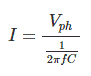So, the capacitive current through the faulty path during single phase to earth fault is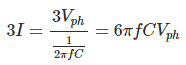After fault, the star point will have phase voltage as the null point is shifted to fault point. So the voltage appears across the inductor is Vph. Hence, the inductive current through the coil is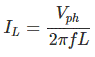Now, for cancellation capacitive current of value 3I, IL must have same magnitude but 180o electrically apart. Therefore,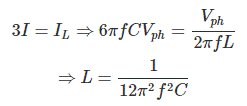When, design or configuration (in length and/or cross section and/or thickness and quality in insulation) of the system changes the inductance of the coil is to be adjusted accordingly. That is why often Petersen coil is provided with tap changing arrangement.

Want To Learn Faster? 🎓
Get electrical articles delivered to your inbox every week.
No credit card required—it’s 100% free.